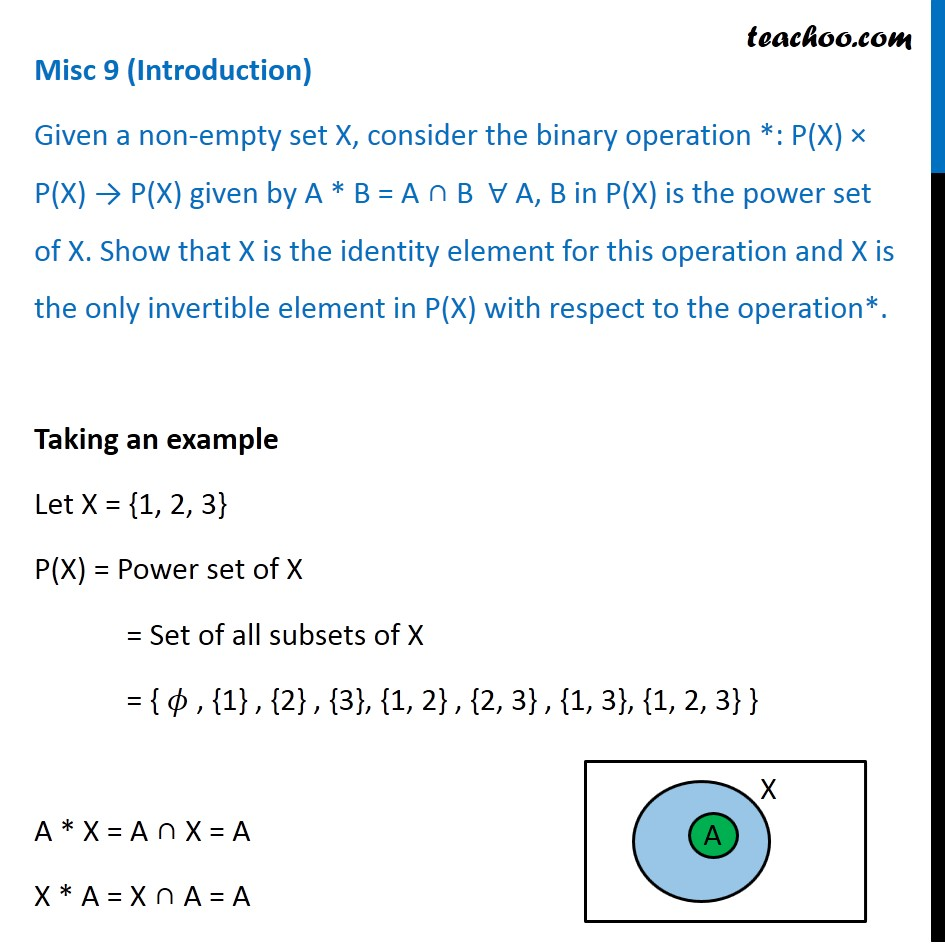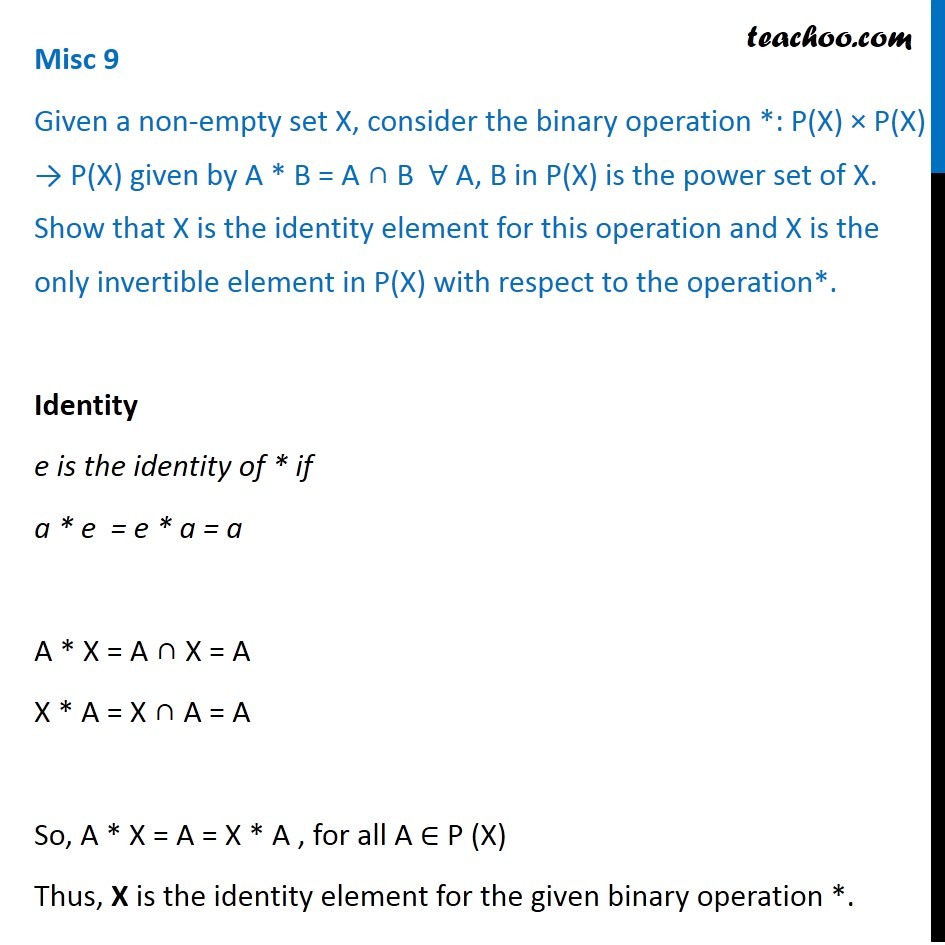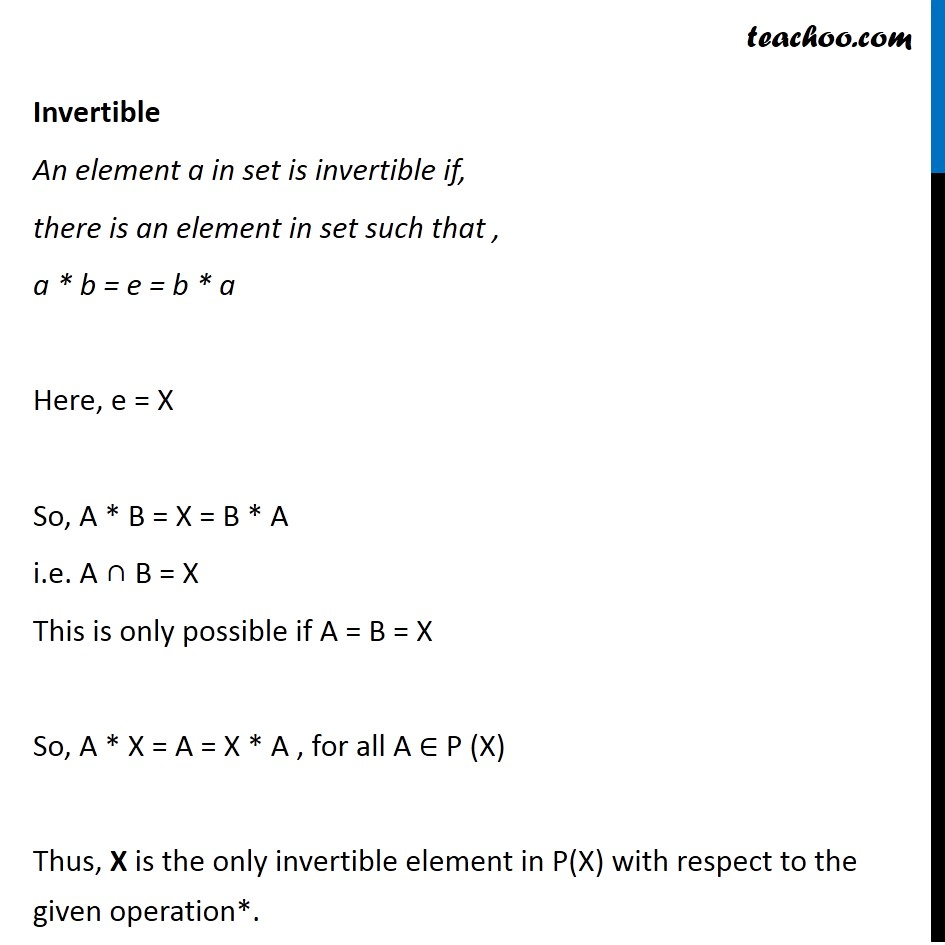Miscellaneous

Chapter 1 Class 12 Relation and Functions
Serial order wiseGet live Maths 1-on-1 Classs - Class 6 to 12

### Transcript

Misc 9 (Introduction) Given a non-empty set X, consider the binary operation *: P(X) × P(X) → P(X) given by A * B = A ∩ B ∀ A, B in P(X) is the power set of X. Show that X is the identity element for this operation and X is the only invertible element in P(X) with respect to the operation*. Taking an example Let X = {1, 2, 3} P(X) = Power set of X = Set of all subsets of X = { 𝜙 , {1} , {2} , {3}, {1, 2} , {2, 3} , {1, 3}, {1, 2, 3} } A * X = A ∩ X = A X * A = X ∩ A = A Misc 9 Given a non-empty set X, consider the binary operation *: P(X) × P(X) → P(X) given by A * B = A ∩ B ∀ A, B in P(X) is the power set of X. Show that X is the identity element for this operation and X is the only invertible element in P(X) with respect to the operation*. Identity e is the identity of * if a * e = e * a = a A * X = A ∩ X = A X * A = X ∩ A = A So, A * X = A = X * A , for all A ∈ P (X) Thus, X is the identity element for the given binary operation *. Invertible An element a in set is invertible if, there is an element in set such that , a * b = e = b * a Here, e = X So, A * B = X = B * A i.e. A ∩ B = X This is only possible if A = B = X So, A * X = A = X * A , for all A ∈ P (X) Thus, X is the only invertible element in P(X) with respect to the given operation*.